# The BasicsDynamics is the name give to the rules of motion. It’s something that you would think would be one of the first things to be figured out, but wasn’t fully locked down until fairly recently. That being said the rules have’t changed much and are fairly predictable, at least on large scales. Someone once told me that all you needed to know for a Dynamics exam was:and everything else could be derived from that. I never found out if they was right, I learnt these ones too, just in case:If you’re already familiar with the equations you might want to skip ahead to the next section, otherwise I’ll explain where they came from and how to use them.

When dealing with measurements you can use scalar or vector quantities.

Scalar quantities:

• Have a magnitude only.
• Energy, Length, Mass, Speed, Temperature and Time are all scalar quantities.

Vector quantities:

• Have both a magnitude and a direction
• Displacement, Force, Velocity, Acceleration and Momentum are all vector quantities.

It might seem sometimes that speed and velocity are the same thing (often they’re equal to each other), but they are actually slightly different. Speed is just how fast something is going, it doesn’t matter if it’s going up, down, left or right, all that matters is how far it travels in a set time. Probably the best way to consider velocity is if you think or an ordinary x,y-axis. If a body is travelling horizontally in a straight line with a speed of 10then stops and goes in the exact opposite direction, with a speed of 10there has obviously been a change, however the speed does not reflect this. The speed before it turned around is the same as after. The velocity however is not the same. If we said that the velocity to begin with was the same as the speed: 10then when the body is travelling in exactly the opposite direction, with the same speed, the velocity would be –10.

# Newtons Laws

Isaac Newton was a clever bloke. We have him to thank for gravity (I should probably add he discovered, not invented it, otherwise people will start blaming him every time they fall over). The things Newton is most famous for (aside from the incident with the apple) are his laws of motion:

1. A particle will remain at rest or continue with its motion, unless acted upon by an external force.
2. The force on an object is equal to its mass multiplied by its acceleration ().
3. Every action has an equal and opposite reaction.

All of this is fine, but what do these laws really mean?

1. A particle will remain at rest or continue with its motion, unless acted upon by an external force.

This just means that provided no external force acts upon a particle it won’t change it’s motion in any way. If there was no friction or air resistance, then a particle moving at 5would carry on indefinitely. Obviously in real life this does not happen as there are air resistance and friction, so it’s almost impossible to have no external force on a moving particle. However, if you think about a stationary particle, this makes far more sense. Unless a force is applied to a stationary particle, it doesn’t start moving.

2. The force on an object is equal to its mass multiplied by its acceleration.

More simply known as, this is probably one of the most fundamental formulae in Dynamics. It’s one of those which pops up all over the place in Dynamics and is a really good idea to learn. It’s not too difficult to understand either. It makes sense that if something has a greater mass, it would take a larger force to give it the same acceleration as something with less mass.

3. Every action has an equal and opposite reaction

This law basically means that if you push against a wall, it pushes you back, which is a good job really because otherwise you’d go straight through!

# Constant Acceleration Formulae

These go by so many different names it’s hard to keep up sometimes. You may have heard them referred to as kinematic equations, equations of motion, SUVAT equations, or maybe you’ve not heard of them at all. First of all lets take a look at them:

(1)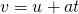(2)(3)(4)(5)There may seem a lot to remember there, but believe me, it’s not as difficult as it seems. Likethese equations are incredibly important in Dynamics.

## SUVAT Equation 1

As you probably already know, velocity divided by time is equal to acceleration and velocity multiplied by time is equal to displacement. This means that on a speed vs. time graph, the gradient of the line is equal to acceleration and the area under the line is equal to displacement.

If you have an initial velocityand a final velocitythe graph would look something like this:

As I previously said, the gradient of the line is equal to acceleration. So. Rearranging this to makethe subject, gives us our first constant acceleration formula:## SUVAT Equation 2

Okay, so one down, just four to go!

We know that the area under the graph is equal to the displacement. So we know thatmultiplied bygives us the bottom rectangle of the area anddivided by 2, gives us the top triangle. This gives us:Now we already know thatso we can rearrange that to giveand then substitute this into our equation for displacement. From this we have. If we just multiply out the bracket that provides us with our second formula:For those of you who like to find maths where they can, you might be interested to know thatis the integral ofwith respect to. If that makes no sense to you, why not go take a look at the wonderful Integration section, where all will become clear!

## SUVAT Equation 3

Now those of you who are keen on spotting patterns may have noticed that this equation looks a lot like the last one. This is because it’s a lot like the last one. Those of you who decided not to go to the Integration page might regret that now.

If you rearrangeto makethe subject you get:Now you just need to integrate this result with respect to time to give you our 3rd equation:## SUVAT Equation 4

We’ve already established that the area under the graph (equal to displacement) is equal to:If we multiply out the bracket we get:which is the same as:Finally we just factorise this to give:## SUVAT Equation 5

We can rearrange, to makethe subject:Then we just substitute this value ofinto our previous equation:, which gives us:which can be simplified to,and thenthis eventually give us the final form ofAnd that’s that! These equations are definitely worth learning since they’re useful time and time again. There are a couple of rules, for instance they can only be used in cases where there is constant acceleration. This means that if the acceleration is something like 12 ms-2 they’re fine, but if the acceleration is along the lines of 12ms-2 then they won’t work since the acceleration varies with.

# What a Drag

A lot of dynamics is done by neglecting air resistance, and while this makes things a lot easier to deal with it’s always worth knowing what affect it would have. For any object moving in a fluid the drag force on it can be calculated using: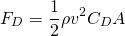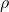is the density of the fluid (998.2071 kg mfor water at 30 degrees and 1.204 kg mfor air),is the velocity of the object,is the objects cross sectional area andis the drag coefficient. The drag coefficient it a number that relates to how aerodynamic the object is, with a cube havingand a sphere havingAn object falling to the Earth it will eventually (if it’s falling for long enough) reach a speed at which the drag force equals the force of gravity pulling it down. This is called Terminal Velocity and you can get an expression for it by equating the drag force toand then rearranging for:For a human falling through the air (from above) we have 70kg, 0.5marea and a drag coefficient of about 0.8 (rough guess somewhere around a angle cube or cylinder) we get a terminal velocity of around 53 m s(which turns out to be a pretty good rough estimate).

# Motion on a flat surface

This is the most simple instance in dynamics. A body moves along a flat surface in a straight line. For example:

1. The Rev is driving his car, when suddenly the engine stops working! If he is travelling at 10 ms-1 and his deceleration is 2 ms-2 how long will it take for the car to come to rest?

Okay, it’s always a good idea with these kind of problems to list what you know. We’re given initial velocity,, and acceleration,. We also know that if the car is going to finish at rest, that final velocity,, must be 0ms-1. We want to find out time,. Personally I find it’s best to lay this information out like this:

u = 10ms-1
v = 0 ms-1
a = -2 ms-2
t = ? s

From this we can see which equation we need. In this case we can see that the equation we want is. We rearrange this to makethe subject, giving us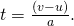Finally, we put the numbers into the equation:.

2. Michael steps into the road, 30 metres from where the engine stops working. The Rev's glasses have fallen off and he does not see Michael. Will The car stop in time to miss hitting Michael?

Once again, it’s best to lay out all the information we have:

u = 10 ms-1
v = 0 ms-1
a = -2 ms-2
t = 5 s
s = ? m

This time we want to find displacement, s, so we need to pick an equation with that in. I’m going to use. I could have used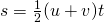or, however, since we’re not given time, but have instead worked it out ourselves, any error made in previous calculations would be carried forward into this one.
Again, I’m going to rearrange the equation, this time to giveas the subject. This is a good habit to get into, it might not make much difference now whether you rearrange the equation before or after putting numbers in, but with more complex formulae it can get really messy if you don’t rearrange it first. Also in exam situations, if you make a mistake, you may still get method marks if the examiner can see what you’ve done.
Anyway, this gives usPutting the numbers into the equation gives us: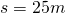, so Michael will not be hit! (Phew!)

In the above example, friction has been completely ignored. In the real world we can’t do this (quite fortunate really because we’d fall over all the time and people would think we were drunk). So now we’d better have a look at a situation with friction. The coefficient of friction is given the symbol μ. The resultant (normal) force of the weight, balances the weight of the car (so it doesn’t go through the road). The force due to friction is μ (or μN).

3. The Rev's car has broken down on the M1. He needs to push it to the hard shoulder. The car weighs 5000N. The Rev can push about 1800N. The coefficient of friction between the car and the road is 0.6. Will The Rev be able to push the car to the hard shoulder?

Okay, so firstly in a situation like this it’s good to draw a little sketch of what’s going on.

From this we know that in order for the car to move, The Rev must be pushing with a force of at least μR. By simply multiplying the coefficient of friction by the resultant force, we find that the force due to friction is 3000N, so The Rev won’t be able to push the car to the side of the road.

4. A body-builder happens to be passing by and in an attempt to ease congestion on the ever busy M1, he decides to help. He can push with a force of 3200N. With both the body-builder and The Rev pushing, what will be the acceleration of the car?
NB – Take the mass of the car to be 510kg

So, same situation as before really, only this time the forces don’t balance and so there will be an acceleration. We get this from the oh-so-clever Isaac Newton’s,.
Remember that to find the overall force you need to take away the frictional force. So that’s (3200 + 1800) – 3000. That leaves an overall force of 2000N. Again we need to rearrange the formula to give a as the subject this time. This gives us. Putting the numbers in we get:a = 3.9 ms-2 (2 s.f.)

# Motion on a Inclined Plane

This is pretty similar to motion on a flat surface, just one or two more variables… oh and we won’t be talking about The Rev’s car any more  since I’m not sure that would make it up a hill!

Anyway, I fear I may have wandered off track a little there. Introducing the “inclined plane”, or “slope” as it’s known to most of us, means you’re going to have to brush up on your trigonometry. On the plus side, you’ll find out why people have been trying to drill it into you for years! Provided you’re familiar with the good old order of operations you should be fine.

The picture above shows us a block sitting on a slope. A good place to start with this (probably the only place to start if you want a chance in hell of getting anywhere with a question) is to resolve forces. Assuming that the block is at rest, we know that it is in equilibrium, so the horizontal forces must be equal and so must the vertical forces (unless it’s one of those lovely levitating blocks).

# Projectile Motion

Projectiles aren’t completely dissimilar to Motion in a straight line, just instead of a body just moving from left to right, it’s going up or down aswell. First lets have a look at a typical example of projectile motion:

A ball is thrown at an an angle of 30°. It has an initial velocity of 20ms-1. Find the maximum height the ball would reach.

Okay so as per usual, we draw a diagram:

Now lets list what we know:

• u = 20 sin30 ms-1
• v = 0 ms-1
• a = -9.81 ms-2
• s = ? m

Now we pick one of the kinematic formulae, the one which is going to get us the result in the most direct way is:, and rearrange it to makethe subject:Then finally put the numbers into the equation: